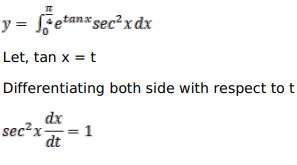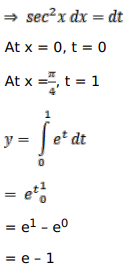# Mark against the correct answer in the following:

Question:

Mark $(\sqrt{)}$ against the correct answer in the following:

$\int_{0}^{\pi / 4} \frac{e^{\tan x}}{\cos ^{2} x} d x=?$

A. $(e-1)$

B. $(\mathrm{e}+1)$

C. $\left(\frac{1}{e}+1\right)$

D. $\left(\frac{1}{e}-1\right)$

Solution: